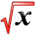# Related ProblemsThis article is part of the MathHelp Tutoring Wiki

Q1: Let R be the region enclosed by the curves y=x+2 and y=x2

(a) find the area of R

(b) find the volume of the solid obtained by rotating R about the line y=-1

Answer: (a)The enclosed region is below the curve of y=x+2, and above the curve of y=-1. So the region is in between.

Using integration along x axis,we can get

Area=\int (x+2-x2)dx   from -1 to 2

=[1/2*x2+2x-1/3*x3] from -1 to 2

=(2+4-8/3)-(1/2-2+1/3)=5-1/2=9/2


(b)We can use disk method to calculate area of each small disk, and then sum them up. For the outer region disk,the radius is x+2+1, for the inner disk, the radius is X2+1. So the area of each disk is the area of the outer disk minus the area of the inner disk.

Volume=\int pi*[(x+2+1)2-(x2+1)2]dx from -1 to 2

=\int pi*(-x4-x2+6x+8)dx from -1 to 2

=pi*[-1/5x5-1/3x3+3x2+8x] from -1 to 2

=pi*(30-33/5)

=117/5*pi

Q2. Find the number b such that the line y=b divides the region bounded by the curves y=x2 and y=4 into two regions with equal area.

Answer:We can interpret the question in this way. The region bounded by y=b and y=x2 is half of the area of region bounded by y=x2 and y=4.

Area of the total region A1

=\int (4-x2) from -2 to 2

=[4x-1/3*x3] from -2 to 2

=16-3/16

=32/3

Curve y=x2 intersects y=b with two points (-sqrt(b),b) and (sqrt(b),b)

Area of the sub-region A2

=\int (b-2) from -sqrt(b) to sqrt(b)

=[bx-1/3*x3] from -sqrt(b) to sqrt(b)

=4/3*b3/2

so A1=2*A2

32/3=8/3*b3/2

b=163/2